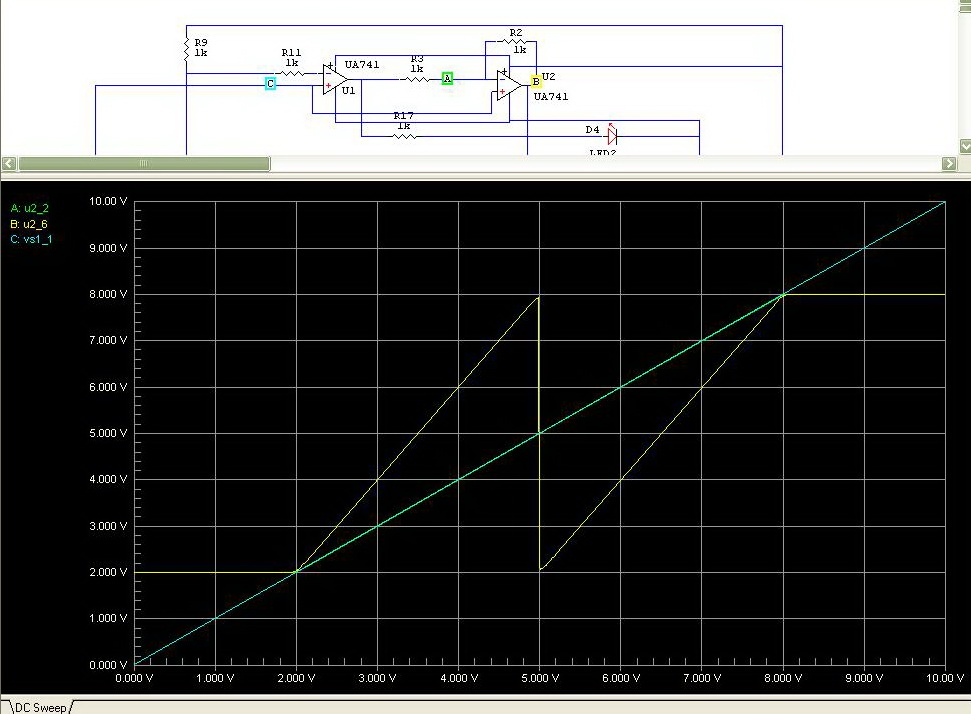# Voltage Level Detector Circuit

## Voltage Level Detector Circuit

This circuit is designed and submitted by Mr. Rakesh Bute. We are really thankful for his contribution.

The circuit as explained by Rakesh:

The design came from the interest of finding a new technique of analog to digital conversion. The two types of ADC (Analog to Digital Converter) that inspired me in the development of this circuit are Flash Type ADC and Successive Approximation Type ADC.  The Flash Type ADC is the fastest ADC available in the market (highest sampling rate 120 Msps) but it uses a huge number of OP-AMPS. On the other hand Successive Approximation Type ADC uses fewer components but its speed is dependent upon the clock frequency provided to it. I was looking for a technique that can give a trade-off between this two, the result of which is this circuit.

### Construction

The working of this circuit is similar to that of decimal fraction to binary fraction conversion. For this purpose, the circuit amplifies a signal and compares it to a reference voltage. The circuit can be divided into a number of stages. Number of stages can be increased or decreased according to need. Each stage contains two OP-AMPs(TL084).  One of them (OP-AMP at the left side) is used for comparison purpose. The other (OP-AMP at the right side) is used as a non-inverting amplifier with a fixed gain (EXACTLY 2). The input voltage is connected to the non-inverting pin/terminal of each OP-AMP. The digital output is obtained from the output of the comparing OP-AMP and the output of the amplifier OP-AMP is fed to the input of the next stage. To obtain a reference voltage, two resistors are used.

### Working

An input voltage is applied. The OP-AMP used as a comparator compares the input voltage with the reference level. If it exceeds a certain reference level, the comparator output goes high and there is amplification along with subtraction operation is performed by the amplifying OP-AMP. If the input voltage is less than the reference voltage, only the amplification operation is performed. The output of the amplifier OP-AMP is inherited to lower stages.

The output of my interest is the outputs obtained from the comparator OP-AMPs. They together represent binary number.

### Circuit DiagramVoltage Level Detector CircuitVoltage Level Detector CircuitSimulation Response

Testing

To see if the circuit is working or not, use a potentiometer, connect its two ends to the two poles of the battery, connect the wiper to the first stage of the circuit. Now as the potentiometer is swiped across its range, a count can be observed represented by the four LED’s. It will be a binary count, either upward or downward.

### Shortcomings

The gain of the amplifier must be 2. Small error in higher stages will deteriorate the performance of lower stages and is un-avoidable since practically a gain, exactly 2 is un-obtainable. Even the 4 stage circuit will show up level loss.

Components

 Count Label-Value PACKAGE Designation 4 LED SIP2 D1,D2,D3,D4 18 1k AXIAL0. R1 to R18 1 10k var SIP3 R19 2 TL084 DIP14 U1,U3 1 20V or 9v V1

Notes

• Any other general purpose OP-AMP can be used in this circuit; Using TL084 reduces the number of connections and makes it simpler.
• Resistors with equal value of resistance can be used. Resistances with value of 1k to 10k can be used. Just they need to have same value. If the tolerance is low, it is good for the circuits.
• There is no need to have a ground connection. Treat it as the negative end of the battery. All the connections to ground are connected to the negative supply of the battery.